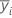# Methods and formulas for the model in One-Way ANOVA

Select the method or formula of your choice.

## Notation

The notation is critical to understanding ANOVA models. Listed below is the notation used for one-way analysis of variance.

TermDescription
r number of levels of the factor, i = 1 ...r
i a given factor level
j a given case for a particular factor level, j = 1 ...n i
yij j th observation of the response for the i th factor level
ni number of observations for the i th factor level
n T total number of cases
μi true mean of observations at the i th factor level
yi. total of the observations at the i th factor levelmean of the response for the ith factor

## Model fitting

### Formula

The one-way analysis of variance model can be specified in several ways. The cell means model is:

All observations for the factor level have the same expectation, μ i . Because μi is a constant, all observations have the same variance, regardless of factor level.

In analysis of variance, least squares estimation is used to fit the model and provide estimates for the parameters, μi .

The hypothesis test for one-way analysis of variance is:

H0: μ 1 = μ 2= … = μ r

H1: At least one mean is not equal to the others

### Notation

TermDescription
μ i parameters or the true mean of observations at the i th factor level
ε ij error that is independently and normally distributed with mean 0 and constant variance σ 2
By using this site you agree to the use of cookies for analytics and personalized content.  Read our policy